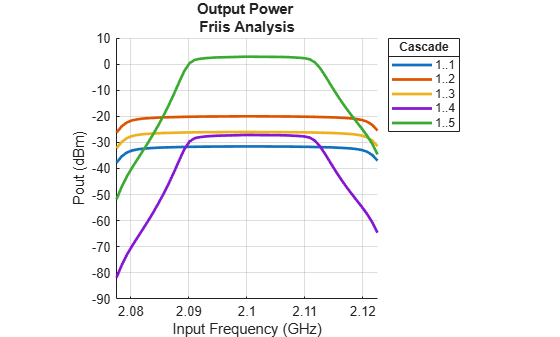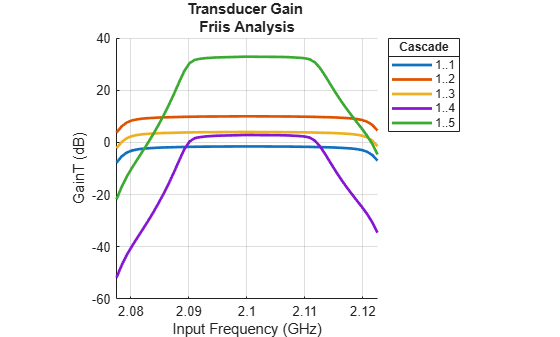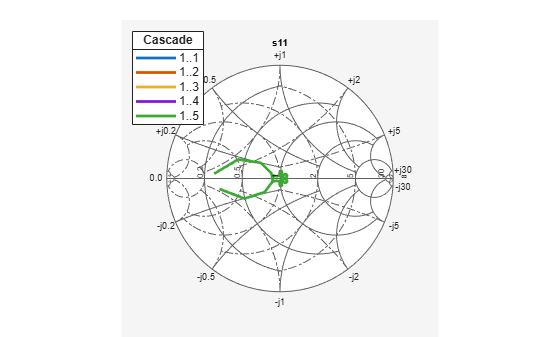# rfbudget

Create RF budget object and compute RF budget results for chain of 2-port elements

## Description

Use the `rfbudget` object to create an rfbudget element to calculate RF budget results of a circuit. You can use any 2-port element in this circuit such as `amplifier`, `nport`, or `modulator`. Open the complete `rfbudget` circuit in an RF Budget Analyzer app. You can also export the completed circuit to RF Blockset™.

## Creation

### Syntax

``rfobj = rfbudget``
``rfobj = rfbudget(elements,inputfreq,inputpwr,bandwidth)``
``rfobj = rfbudget(___,autoupdate)``
``rfobj = rfbudget(...,Name,Value)``

### Description

example

````rfobj = rfbudget` creates an `rfbudget` object, `rfobj`, with default empty property values.```

example

````rfobj = rfbudget(elements,inputfreq,inputpwr,bandwidth)` creates an RF budget object from the input RF elements, and independently computes an RF budget analysis at the specified input frequencies, available input power, and signal bandwidth. The input arguments are stored in the `Elements`, `InputFrequency`, `AvailableInputPower`, and `SignalBandwidth` properties. The analysis results are stored in dependent properties. By default, if any of the input properties are changed, the object recomputes results.```
````rfobj = rfbudget(___,autoupdate)`sets the `AutoUpdate` property. Setting `AutoUpdate` to false turns off automatic budget recomputation as parameters are changed. You can use this syntax with any of the previous syntaxes.```
````rfobj = rfbudget(...,Name,Value)`creates RF budget object with additional properties specified by one or more name-value pair arguments. `Name` is the property name and `Value` is the corresponding value. You can specify several name-value pair arguments in any order as `Name1`, `Value1`, `...`, `NameN`, `ValueN`. Properties not specified retain their default values.```

## Properties

expand all

RF budget elements, specified as the comma-separated pair consisting of `'Elements'` and an RF toolbox object or array of RF toolbox objects. The possible elements are `amplifier`, `modulator`, generic `rfelement`, and `nport` objects. To specify a circuit consisting of multiple RF objects, specify the elements as a cell array. For information on edge cases, see Algorithms.

Example: ```a = amplifier;m = modulator;rfbudget('Elements',[a m])``` calculates the RF budget analysis of the amplifier and modulator circuit.

Input frequency of signal, specified as the comma-separated pair consisting of `'InputFrequency'` and a scalar or vector in Hz. If the input frequency is a vector, then the RF budget object calculates the analysis for each input frequency separately.

Example: `'InputFrequency',2e9`

Data Types: `double`

Power applied at the input of the cascade, specified as the comma-separated pair consisting of `'AvailableInputPower'` and a scalar in dBm.

Example: `'AvailableInputPower',-30`

Data Types: `double`

Signal bandwidth at the input of the cascade, specified as the comma-separated pair consisting of `'SignalBandwidth'` and a scalar in Hz.

Example: `'SignalBandwidth',10`

Data Types: `double`

Option to automatically recompute the RF budget analysis by incorporating changes made to the existing circuit, specified as the comma-separated pair consisting of `'AutoUpdate'` and a boolean scalar.

Example: `'AutoUpdate',true`

Data Types: `logical`

Computation method, specified as `Friis` or `HarmonicBalance`. The `Friis` solver is faster than the `HarmonicBalance` solver, but does not support computation of nonlinearities such as OIP2. The `HarmonicBalance` solver, the tone and harmonic-dependent properties are displayed.

Note

`HarmonicBalance` does not support architectures where the input or output frequencies at any stage are nonzero and less than `SignalBandwidth`.

Example: `'Solver','Friis'`

Data Types: `string`

Output frequencies, specified as the comma-separated pair consisting of `'OutputFrequency'` and a row vector in Hz.

Data Types: `double`

Output power, specified as the comma-separated pair consisting of `'OutputPower'` and a row vector in dBm.

Data Types: `double`

Transducer power gains, specified as the comma-separated pair consisting of `'TransducerGain'` and a row vector in dB.

Data Types: `double`

Noise figures, specified as the comma-separated pair consisting of `'NF'` and a row vector in dB.

Data Types: `double`

Input-referred second-order intercept, specified as the comma-separated pair consisting of `'IIP2'` and a row vector in dBm. These values are computed only when the selected solver is `HarmonicBalance` solver.

Data Types: `double`

Output-referred second-order intercept, specified as the comma-separated pair consisting of `'OIP2'` and a row vector in dBm. These values are computed only when the selected solver is `HarmonicBalance` solver.

Data Types: `double`

The Output-referred third-order intercept, specified as the comma-separated pair consisting of `'OIP3'` and a row vector in dBm.

Data Types: `double`

The Input-referred third-order intercept, specified as the comma-separated pair consisting of `'IIP3'` and a row vector in dBm.

Data Types: `double`

Signal-to-noise ratio, specified as the comma-separated pair consisting of `'SNR'` and a row vector in dB.

Data Types: `double`

Displays progress bar with cancel button during harmonic balance analysis, specified as `true` or `false`.

Data Types: `logical`

## Object Functions

 `show` Graphical summary of all relevant spurs and spur-free zones `computeBudget` Compute results of rfbudget object `exportScript` Export MATLAB code that generates RF budget object `exportRFBlockset` Create RF Blockset model from RF budget object `exportTestbench` Create measurement testbench from RF budget object `rfplot` Plot cumulative RF budget result versus cascade input frequency `smithplot` Plot impedance transformation for selected matching network on smith chart `polar` Plot specified object parameters on polar coordinates

## Examples

collapse all

Open a default RF budget object.

`obj = rfbudget`
```obj = rfbudget with properties: Elements: [] InputFrequency: [] Hz AvailableInputPower: [] dBm SignalBandwidth: [] Hz Solver: Friis AutoUpdate: true ```

Create an amplifier with a gain of 4 dB.

`a = amplifier('Gain',4);`

Create a modulator with an OIP3 of 13 dBm.

`m = modulator('OIP3',13);`

Create an nport using `passive.s2p`.

`n = nport('passive.s2p');`

Create an rf element with a gain of 10 dB.

`r = rfelement('Gain',10);`

Calculate the rf budget of a series of rf elements at an input frequency of 2.1 GHz, an available input power of -30 dBm, and a bandwidth of 10 MHz.

`b = rfbudget([a m r n],2.1e9,-30,10e6)`
```b = rfbudget with properties: Elements: [1x4 rf.internal.rfbudget.Element] InputFrequency: 2.1 GHz AvailableInputPower: -30 dBm SignalBandwidth: 10 MHz Solver: Friis AutoUpdate: true Analysis Results OutputFrequency: (GHz) [ 2.1 3.1 3.1 3.1] OutputPower: (dBm) [ -26 -26 -16 -20.6] TransducerGain: (dB) [ 4 4 14 9.4] NF: (dB) [ 0 0 0 0.1392] IIP2: (dBm) [] OIP2: (dBm) [] IIP3: (dBm) [ Inf 9 9 9] OIP3: (dBm) [ Inf 13 23 18.4] SNR: (dB) [73.98 73.98 73.98 73.84] ```

Show the analysis in the RF Budget Analyzer app.

`show(b)`Create an RF system.

Create an RF bandpass filter using the Touchstone® file `RFBudget_RF`.

`f1 = nport('RFBudget_RF.s2p','RFBandpassFilter');`

Create an amplifier with a gain of 11.53 dB, a noise figure (NF) of 1.53 dB, and an output third-order intercept (OIP3) of 35 dBm.

`a1 = amplifier('Name','RFAmplifier','Gain',11.53,'NF',1.53,'OIP3',35);`

Create a demodulator with a gain of -6 dB, a NF of 4 dB, and an OIP3 of 50 dBm.

```d = modulator('Name','Demodulator','Gain',-6,'NF',4,'OIP3',50, ... 'LO',2.03e9,'ConverterType','Down');```

Create an IF bandpass filter using the Touchstone file `RFBudget_IF`.

`f2 = nport('RFBudget_IF.s2p','IFBandpassFilter');`

Create an amplifier with a gain of 30 dB, a NF of 8 dB, and an OIP3 of 37 dBm.

`a2 = amplifier('Name','IFAmplifier','Gain',30,'NF',8,'OIP3',37);`

Calculate the RF budget of the system using an input frequency of 2.1 GHz, an input power of -30 dBm, and a bandwidth of 45 MHz.

`b = rfbudget([f1 a1 d f2 a2],2.1e9,-30,45e6)`
```b = rfbudget with properties: Elements: [1x5 rf.internal.rfbudget.Element] InputFrequency: 2.1 GHz AvailableInputPower: -30 dBm SignalBandwidth: 45 MHz Solver: Friis AutoUpdate: true Analysis Results OutputFrequency: (GHz) [ 2.1 2.1 0.07 0.07 0.07] OutputPower: (dBm) [-31.53 -20 -26 -27.15 2.847] TransducerGain: (dB) [-1.534 9.996 3.996 2.847 32.85] NF: (dB) [ 1.533 3.064 3.377 3.611 7.036] IIP2: (dBm) [] OIP2: (dBm) [] IIP3: (dBm) [ Inf 25 24.97 24.97 4.116] OIP3: (dBm) [ Inf 35 28.97 27.82 36.96] SNR: (dB) [ 65.91 64.38 64.07 63.83 60.41] ```

Plot the available output power.

```rfplot(b,'Pout') view(90,0)```Plot the transducer gain.

```rfplot(b,'GainT') view(90,0)```Plot S-parameters of an RF system on a Smith Chart and a Polar plot.

`s = smithplot(b,1,1,'GridType','ZY'); ``p = polar(b,2,1); `Create two modulators, m1 and m2, with output-referred second-order intercept set to 20 and available power gain set to 3.

```m = modulator('Gain',3,'OIP2',20,'ImageReject',false,'ChannelSelect',false); m2 = modulator('Gain',3,'OIP2',20,'ImageReject',false,'ChannelSelect',false);```

Create a RF budget object specifying the input frequency of the signal, power applied at cascade, and signal bandwidth. Select `HarmonicBalance` as solver method to compute nonlinear effects such as IIP2 and OIP2.

`b = rfbudget([m m2],2.1e9,-30,100e6,'Solver','HarmonicBalance')`
```b = rfbudget with properties: Elements: [1x2 modulator] InputFrequency: 2.1 GHz AvailableInputPower: -30 dBm SignalBandwidth: 100 MHz Solver: HarmonicBalance WaitBar: true AutoUpdate: true Analysis Results OutputFrequency: (GHz) [ 3.1 4.1] OutputPower: (dBm) [ -27 -24] TransducerGain: (dB) [ 3 6] NF: (dB) [ 3.01 7.783] IIP2: (dBm) [ 17 4.457] OIP2: (dBm) [ 20 10.46] IIP3: (dBm) [ Inf Inf] OIP3: (dBm) [ Inf Inf] SNR: (dB) [60.96 56.19] ```

Create an amplifier with a gain of 4 dB.

`a = amplifier('Gain',4);`

Create a modulator with an OIP3 of 13 dBm.

`m = modulator('OIP3',13);`

Create an nport using `passive.s2p`.

`n = nport('passive.s2p');`

Create an rf element with a gain of 10 dB.

`r = rfelement('Gain',10);`

Calculate the rf budget of a series of rf elements at an input frequency of 2.1 GHz, an available input power of -30 dB, and a bandwidth of 10 MHz.

`b = rfbudget([a m r n],2.1e9,-30,10e6);`

Show the analysis in the RF Budget Analyzer app.

`rfplot(b)`Group Delay

To plot the group delay, first plot the S11 data for the RF System.

`rfplot(b,1,1)`Use the `Group Delay `option on the plot graph to plot the group delay of the RF system.Phase Delay

Use the `Phase Delay` option on the plot graph to plot the phase delay of the RF System.## Algorithms

expand all

ABCD parameters are used in the computation of S-parameters of the cascade for Friis Solver. When S21 = 0, conversion to ABCD results in NaNs. For such cases, modifications to the S-parameters are made as follows: